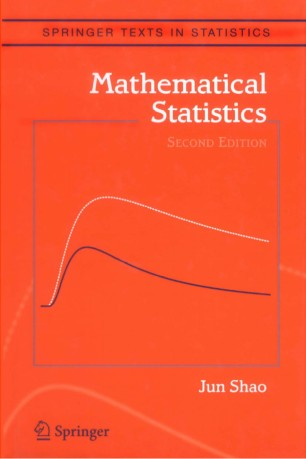# Mathematical StatisticsTextbook

Part of the Springer Texts in Statistics book series (STS)

1. Front Matter
Pages i-xvi
2. Pages 1-90
3. Pages 91-160
4. Pages 161-230
5. Pages 231-318
6. Pages 319-392
7. Pages 393-470
8. Pages 471-542
9. Back Matter
Pages 543-593

### Introduction

This graduate textbook covers topics in statistical theory essential for graduate students preparing for work on a Ph.D. degree in statistics. The first chapter provides a quick overview of concepts and results in measure-theoretic probability theory that are useful in statistics. The second chapter introduces some fundamental concepts in statistical decision theory and inference. Chapters 3-7 contain detailed studies on some important topics: unbiased estimation, parametric estimation, nonparametric estimation, hypothesis testing, and confidence sets. A large number of exercises in each chapter provide not only practice problems for students, but also many additional results.

In addition to improving the presentation, the new edition makes Chapter 1 a self-contained chapter for probability theory with emphasis in statistics. Added topics include useful moment inequalities, more discussions of moment generating and characteristic functions, conditional independence, Markov chains, martingales, Edgeworth and Cornish-Fisher expansions, and proofs to many key theorems such as the dominated convergence theorem, monotone convergence theorem, uniqueness theorem, continuity theorem, law of large numbers, and central limit theorem. A new section in Chapter 5 introduces semiparametric models, and a number of new exercises were added to each chapter.

### Keywords

Mathematical Statistics Statistical Theory likelihood Markov chain Mathematica mathematical statistics probability probability theory statistical theory statistics

### Bibliographic information

• Book Title Mathematical Statistics
• Authors Jun Shao
• Series Title Springer Texts in Statistics
• DOI https://doi.org/10.1007/b97553
• Copyright Information Springer-Verlag New York 2003
• Publisher Name Springer, New York, NY
• eBook Packages
• Hardcover ISBN 978-0-387-95382-3
• Softcover ISBN 978-1-4419-2978-5
• eBook ISBN 978-0-387-21718-5
• Series ISSN 1431-875X
• Edition Number 2
• Number of Pages XVI, 592
• Number of Illustrations 0 b/w illustrations, 0 illustrations in colour
• Topics
• Buy this book on publisher's site

## Reviews

From the reviews of the second edition:

"The second edition of Mathematical Statistics  continues to hold its identity among many other available books on mathematical statistics...The revised and updated version remains of high quality, and I recommend it for use as a text or reference book in a graduate statistics program." Journal of the American Statistical Association, September 2004

"The first edition of this book was published in 1999 … . The main changes include addition of new material in Chapter 1, addition and deletion of a number of exercises, addition of two new sub-sections … . The book remains valuable to instructors and graduate students of traditional mathematical statistics courses, specially for its large collection of problems and for its rigourous presentation." (Arup Bose, Sankhya: The Indian Journal of Statistics, Vol. 65 (3), 2003)

"This book is intended for an advanced postgraduate course in Mathematical Statistics, offered in a mathematically rigorous fashion. … in order to get to grips with rigorous mathematical statistics, this is an ideal book. Also, as a reference book, it is ideally suited. … Two particularly attractive features of the book are the large number of exercises at the end of each chapter – well over a hundred in each chapter, and the fact that asymptotic theory is studied throughout the book … ." (Tertius de Wet, SASJ – South African Statistical Journal, March, 2004)